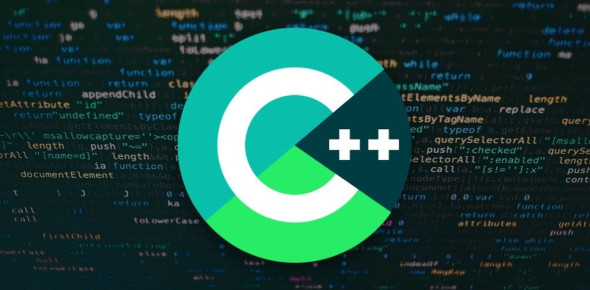# C++ Quiz 2

17 Questions | Total Attempts: 787Settings.

• 1.
Which of the following is not a valid identifier?
• A.

Return

• B.

MyInt

• C.

MyInteger

• D.

Total3

• 2.
What is the value of x after the following statements? int x; x = 0; x = x + 30;
• A.

0

• B.

30

• C.

33

• D.

Garbage

• 3.
What is the output of the following code? float value; value = 33.5; cout << "value" << endl;
• A.

33.5

• B.

33

• C.

Value

• D.

Garbage

• 4.
Which of the following lines correctly reads a value from the keyboard and stores it in the variable named myFloat?
• A.

Cin >> myFloat;

• B.

Cin

• C.

Cin >> "myFloat";

• D.

Cin >> myFloat >> endl;

• 5.
Another way to write the value 3452211903 is
• A.

3.452211903e09

• B.

3.452211903e-09

• C.

3.452211903x09

• D.

3452211903e09

• 6.
What is the value of x after the following statements? float x; x = 15/4;
• A.

3.75

• B.

4.0

• C.

3.0

• D.

60

• 7.
What is the value of x after the following statements? int x; x = 15 %4;
• A.

15

• B.

4

• C.

3

• D.

3.75

• 8.
What is the value of x after the following statement? float x; x = 3.0 / 4.0 + 3  + 2 / 5
• A.

5.75

• B.

4.75

• C.

1.75

• D.

3.75

• 9.
What is the value of x after the following statements? double x; x = 0; x += 3.0 * 4.0; x -= 2.0;
• A.

22.0

• B.

12.0

• C.

10.0

• D.

14.0

• 10.
If x has the value of 3, y has the value of -2, and w is 10, is the following condition true or false? if( x < 2 && w < y)
• A.

True

• B.

False

• 11.
What is the correct way to write the condition y < x < z?
• A.

(y < x < z)

• B.

((y < x) && z)

• C.

((y > x) || (y < z))

• D.

((y < x) && (x < z))

• 12.
Given the following code fragment, what is the output? int x=5; if( x > 5)     cout << "x is bigger than 5. ";     cout <<"That is all. "; cout << "Goodbye\n";
• A.

X is bigger than 5. That is all

• B.

X is bigger than 5

• C.

That is all. Goodbye

• D.

Goodbye

• 13.
Executing one or more statements one or more times is known as:
• A.

Selection

• B.

Iteration

• C.

Sequence

• D.

Algorithm

• 14.
Given the following code fragment, what is the final value of y? int x, y; x = -1; y = 0; while(x <= 3) {     y += 2;     x += 1; }
• A.

2

• B.

10

• C.

6 6

• D.

8

Related TopicsBack to top